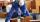# Pears

There were pears in the basket, I took two-fifths of them, and left six in the basket. How many pears did I take?

Result

x =  4

#### Solution:

h - x = 6
x = 2/5h

h-x = 6
2h-5x = 0

h = 10
x = 4

Calculated by our linear equations calculator.

Leave us a comment of example and its solution (i.e. if it is still somewhat unclear...):

Showing 0 comments:Be the first to comment!#### To solve this verbal math problem are needed these knowledge from mathematics:

Need help calculate sum, simplify or multiply fractions? Try our fraction calculator. Do you have a linear equation or system of equations and looking for its solution? Or do you have quadratic equation?

## Next similar examples:

1. TreesFrom the total number of trees in the orchard, there are two-fifths pearls and apples are three eighty. The rest of the trees are 9 ceremonial. How many trees are in the set?
2. Boys and girlsThere are 28 girls in the hall. 5/7 of all children are boys. How many children and how many boys are there?
3. The bunsKate, Zofia and Peter Liked buns. Even today, their grandmother prepare their favorite meal. Katka eats 4 bunches, Žofia 3 and Petra eats 5 buns. Their grandmother said to them, "My inmate will you know how many buns I have been make today, if those you ea
4. ClassroomOne-eighth of 9th class was interested in studying at a grammar school, at a business academy one sixth, at secondary vocational schools quarter, to SOU one third and the remaining three students were interested in the school of art direction. How many st
5. Grandmother and grandfatherGrandmother baked cakes. Grandfather ate half, then quarter of the rest ate Peter and Paul ate half of rest. For parents left 6 cakes. How many cakes maked the grandmother?
6. Bricklayers8 bricklayers build a house for 630 days. How many bricklayers have to add after 150 days, so then the whole building completed in (next) 320 days?
7. EqnSolve equation with fractions: 2x/3-50=40+x/4
8. Unknown numberI think the number - its sixth is 3 smaller than its third.
9. Equation with fractionsSolve equation: ? It is equation with fractions.
10. Unknown number 6Determine the unknown number, which is by 1.5 greater than its fourth.
11. FractionsThree-quarters of an unknown number are 4/5. What is 5/6 of this unknown number?
12. EquationSolve equation and check the result: 1.4x - 3/2 + x - 9,8 = x + 0,4/3 - 7 + 1,6/6
13. NumberI think the number. If I add to its third seven I get same as when to its quarter add 8. Which is the number?
14. UN 1If we add to an unknown number his quarter, we get 210. Identify unknown number.
15. EquationFind x: x + 1/2 = 1/3
16. Unknown number 6Determine x if 1/6 of x is equal to 2/5 of the number 24.
17. Simple equationSolve for x: 3(x + 2) = x - 18